Latest Banking jobs   »

# Quantitative Aptitude Quiz For IRDA AM 2023- 13th April

Direction (1-5) Given below is the pie chart which shows the number of persons visiting a national park on different days and table shows the ratio of male to female visiting these parks.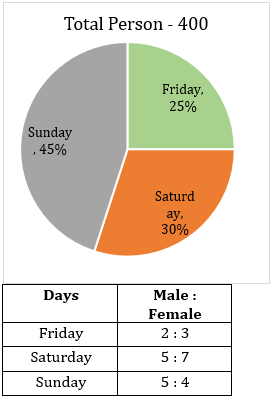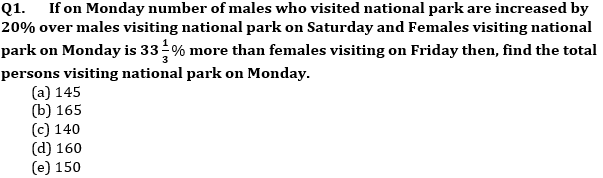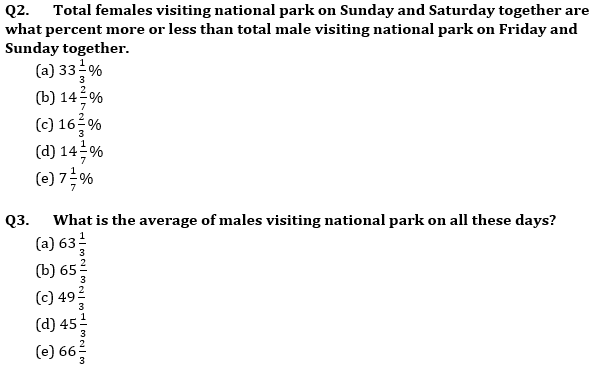Q4. If cost of ticket per male and per female on any day is Rs 45 and Rs 40 respectively then total amount obtained by national park on Friday is how much more or less than total amount obtained by national park on Sunday (in Rs).
(a) 2800
(b) 3500
(c) 3000
(d) 3200
(e) 4200

Q5. What is the ratio of males visiting park on Friday to females visiting park on Saturday?
(a) 4 : 5
(b) 7 : 3
(c) 3 : 7
(d) 4 : 7
(e) 7 : 4

Q6. Four years hence, sum of ages of A and B will be 16 years more than the sum of present age of B and C. Four years ago, sum of age of A and C is 32 years then find the present age of C?
(a) 24 years
(b) 20 years
(c) 12 years
(d) 16 years
(e) 18 years

Q7. A person purchased two articles at the same price and on selling the first article he makes a profit of 12%. Selling price of second article is Rs 90 more than the selling price of the first article. Find the cost price of one article if his overall profit percent is 15%?
(a) Rs 1800
(b) Rs 1500
(c) Rs 2000
(d) Rs 2400
(e) None of these

Q8. Ratio of speed of boat in still water to the speed of current is 10:1. Ratio of time taken by the boat to cover D km in downstream to the time taken by the boat to cover (D-45) km in upstream is 3:2. Then find the value of D?
(a) 60 km
(b) 87 km
(c) 99 km
(d) 108 km
(e) 90 km

Q9. A container contains a mixture of milk and water in which water is 24%. 50% of the mixture is taken out in which water is 78 litres less than the milk. Find the remaining quantity of milk in that container?
(a) 171 lit
(b) 152 lit
(c) 133 lit
(d) 108 lit
(e) 114 lit

Q10. The perimeter of a triangle is equal to perimeter of a rectangle. Length of rectangle is 75% of side of a square and ratio of length to breadth of rectangle is 3 : 2. If difference between perimeter of square and that of rectangle is 36 cm, then find perimeter of triangle?
(a) 60 cm
(b) 48 cm
(c) 72 cm
(d) 80 cm
(e) 96 cm

Direction (11– 15): Solve the given quadratic equations and mark the correct option based on your answer.
(a) if x>y
(b) if x≥y
(c) if x<y
(d) if x ≤y
(e) if x = y or no relation can be established between x and y.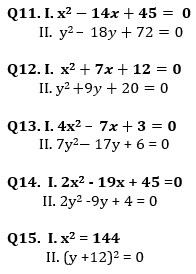Solutions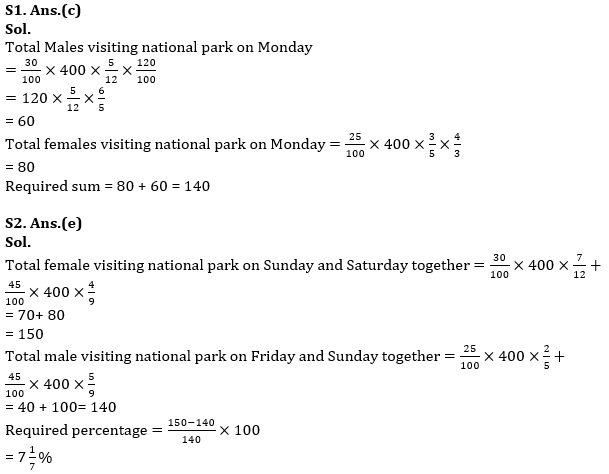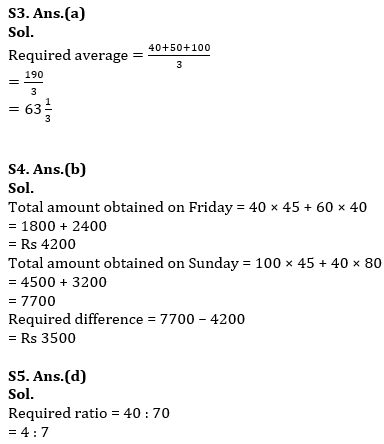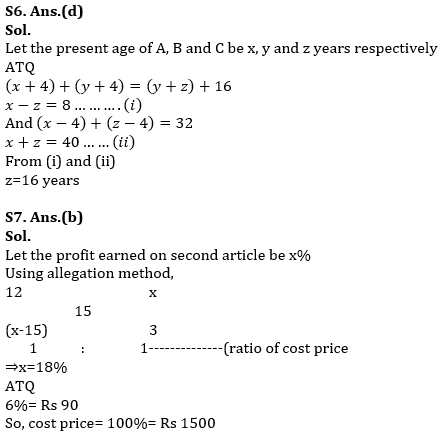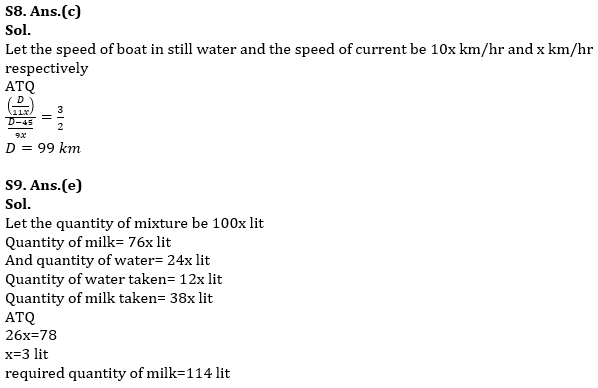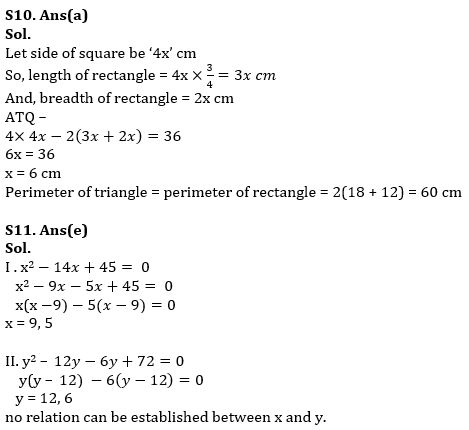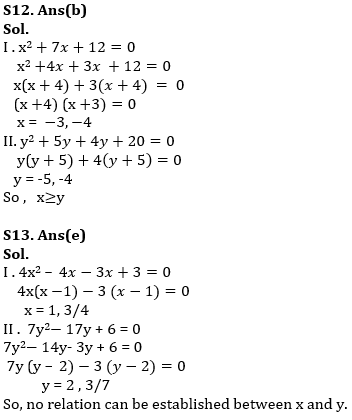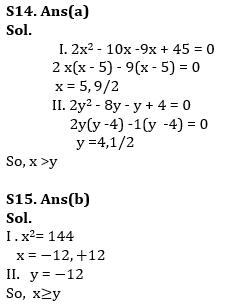## FAQs

#### Congratulations!Union Budget 2023-24: Free PDF